# How to use the QUARTILE function in Excel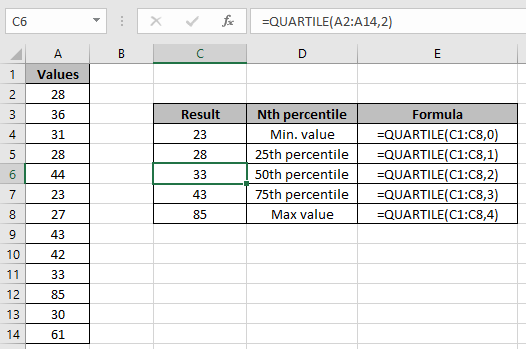In this article, we will learn How to use the QUARTILE function in Excel.

Quartile of a number

The group of quarters which is 1/4th of the total. For example, consider 200 total marks. First quartile is between 0 and 50 (25% of the 200). Second quartile is between 51 and 100. Third percentile is between 101 and 150 and the last fourth quartile is between 151 and 200. Students scoring in these ranges will be grouped under that quartile.

You can extract the exact percentile borders and apply required steps. Let's learn the QUARTILE function and illustrate the function using an example

QUARTILE Function in Excel

Quartile function returns the nth percentile of the range of values.

It returns minimum value, 1/4th quartile value, 1/2th quartile value, 3/4th value & maximum value using the quart argument.

QUARTILE Function Syntax:

 =QUARTILE(array, quart)

array : list of scores given as array reference. Like C2:C18

quart : a number showing the percentile value

 Quart value Nth percentile 0 0th percentile or Min Value 1 25th percentile 2 50th percentile 3 75th percentile 4 100th percentile or Max Value

Example :

All of these might be confusing to understand. Let's understand how to use the function using an example. Here

The nth percentile value for the range of values : 0,1,2,3,4

Use the formula:

 =QUARTILE(H2:H6,0)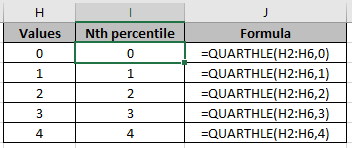The red cells are the negative numbers provided in different formatting styles.

Now we wish to find the quartile of the data set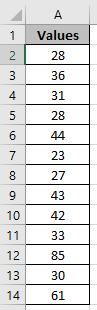Use the formula

 =QUARTILE(A2:A14,0)As you can see the quartile of the range using the Excel Quartile function.

Difference with the percentile function

PERCENTILE function calculates the exact percentile depending upon the percentile value.

Here we have a set of values as data ( A1 : A23 ) and we need to find some percentile values from the array.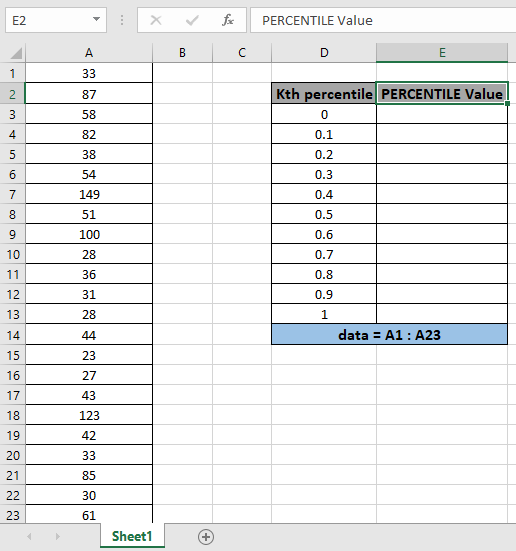Use the formula:

 = PERCENTILE ( data , D3)

data - array given using named range.

D3 - value of k given as cell reference.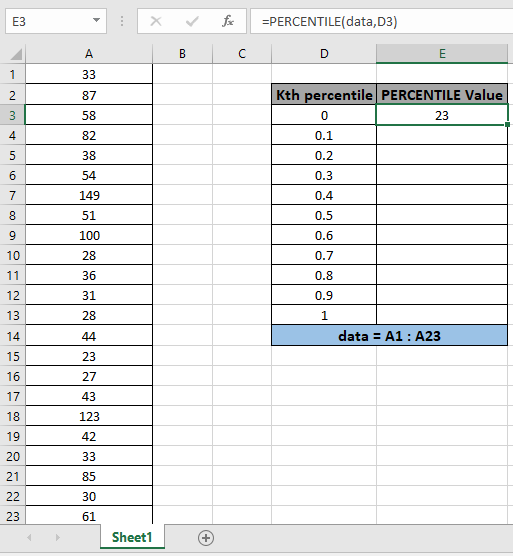The function returns the 0th percentile value or minimum value which is 28 .

Now copy the formula to get different percentile values of the range to the remaining cells and formula as shown in the snapshot below.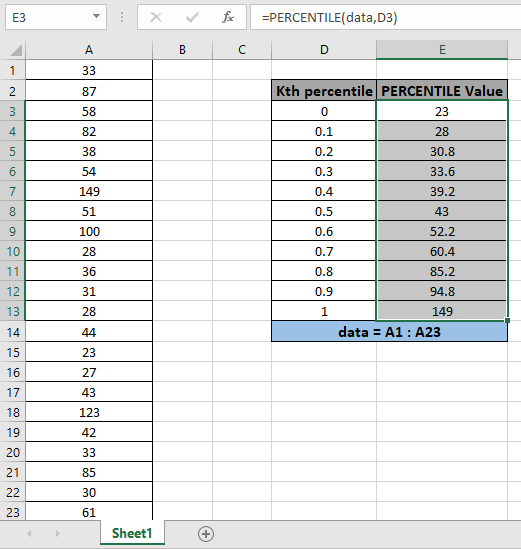As you can see the results in the snapshot above.

Here are all the observational notes using the QUARTILE function in Excel
Notes :

1. The function returns #VALUE! Error if the argument to the function is non numeric.
2. The function returns #NUM! error if the value of k is less than equal to zero or greater than 1.
3. The array argument to the function ignores text, Logic values or Date & Time values.

Hope this article about How to use the QUARTILE function in Excel is explanatory. Find more articles on calculating values and related Excel formulas here. If you liked our blogs, share it with your friends on Facebook. And also you can follow us on Twitter and Facebook. We would love to hear from you, do let us know how we can improve, complement or innovate our work and make it better for you. Write to us at info@exceltip.com.

Related Articles :

How to use the PERCENTILE function in Excel

How to use the PERCENTILE.INC function in Excel

How to use the PERCENTILE.EXC function in Excel

How to use the PERCENTRANK function in Excel

How to use the PERCENTRANK.INC function in Excel

How to use the PERCENTRANK.EXC function in Excel

How to use the QUARTILE function in Excel

How to use the QUARTILE.INC function in Excel

How to use the QUARTILE.EXC function in Excel

Popular Articles :

How to use the IF Function in Excel : The IF statement in Excel checks the condition and returns a specific value if the condition is TRUE or returns another specific value if FALSE.

How to use the VLOOKUP Function in Excel : This is one of the most used and popular functions of excel that is used to lookup value from different ranges and sheets.

How to use the SUMIF Function in Excel : This is another dashboard essential function. This helps you sum up values on specific conditions.

How to use the COUNTIF Function in Excel : Count values with conditions using this amazing function. You don't need to filter your data to count specific values. Countif function is essential to prepare your dashboard.

Terms and Conditions of use

The applications/code on this site are distributed as is and without warranties or liability. In no event shall the owner of the copyrights, or the authors of the applications/code be liable for any loss of profit, any problems or any damage resulting from the use or evaluation of the applications/code.